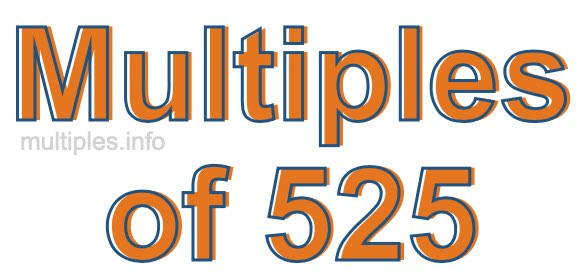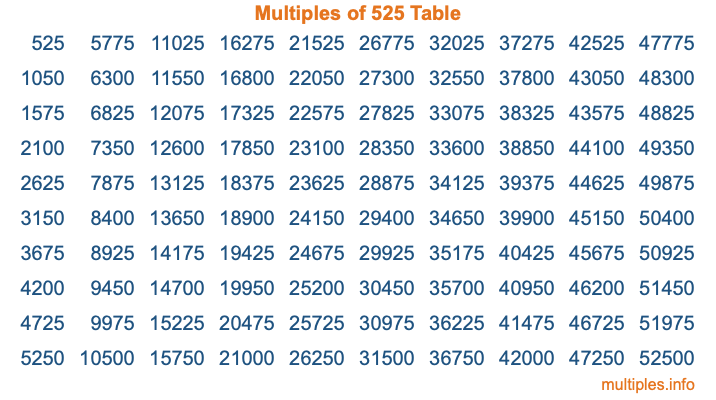Multiples of 525Welcome to the Multiples of 525 page. Here we will first teach you everything you will ever need to know about the multiples of 525, and then give you a study guide summary of everything we taught you to make sure you remember it all. Use this page to look up facts and learn information about the multiples of 525. This page will make you a multiples of five hundred twenty-five expert!

Definition of Multiples of 525
Multiples of 525 are all the numbers that when divided by 525 equal an integer. Each of the multiples of 525 are called a multiple. A multiple of 525 is created by multiplying 525 by an integer.

Therefore, to create a list of multiples of 525, you start with 1 multiplied by 525, then 2 multiplied by 525, then 3 multiplied by 525, and so on for as long as you want. Thus, the list of the first five multiples of 525 is 525, 1050, 1575, 2100, and 2625. To see a larger list of multiples of 525, see the printable image of Multiples of 525 further down on this page. We also have a category where you can choose any nth multiple of 525.

Multiples of 525 Checker
The Multiples of 525 Checker below checks to see if any number of your choice is a multiple of 525. In other words, it checks to see if there is any number (integer) that when multiplied by 525 will equal your number. To do that, we divide your number by 525. If the the quotient is an integer, then your number is a multiple of 525.

Is  a multiple of 525?

Least Common Multiple of 525 and ...
A Least Common Multiple (LCM) is the lowest multiple that two or more numbers have in common. This is also called the smallest common multiple or lowest common multiple and is useful to know when you are adding our subtracting fractions. Enter one or more numbers below (525 is already entered) to find the LCM.

Check out our LCM Calculator if you need more details about the Least Common Multiple or if you need the LCM for different numbers for adding and subtraction fractions.

nth Multiple of 525
As we stated above, 525 is the first multiple of 525, 1050 is the second multiple of 525, 1575 is the third multiple of 525, and so on. Enter a number below to find the nth multiple of 525.

th multiple of 525

Multiples of 525 vs Factors of 525
525 is a multiple of 525 and a factor of 525, but that is where the similarities end. All postive multiples of 525 are 525 or greater than 525. All positive factors of 525 are 525 or less than 525.

Below is the beginning list of multiples of 525 and the factors of 525 so you can compare:

Multiples of 525: 525, 1050, 1575, 2100, 2625, etc.

Factors of 525: 1, 3, 5, 7, 15, 21, 25, 35, 75, 105, 175, 525

As you can see, the multiples of 525 are all the numbers that you can divide by 525 to get a whole number. The factors of 525, on the other hand, are all the whole numbers that you can multiply by another whole number to get 525.

It's also interesting to note that if a number (x) is a factor of 525, then 525 will also be a multiple of that number (x).

Multiples of 525 vs Divisors of 525
The divisors of 525 are all the integers that 525 can be divided by evenly. Below is a list of the divisors of 525.

Divisors of 525: 1, 3, 5, 7, 15, 21, 25, 35, 75, 105, 175, 525

The interesting thing to note here is that if you take any multiple of 525 and divide it by a divisor of 525, you will see that the quotient is an integer.

Multiples of 525 Table
Below is an image of the first 100 multiples of 525 in a table. The table is in chronological order, column by column. The first column has the first ten multiples of 525, the second column has the next ten multiples of 525, and so on.The Multiples of 525 Table is also referred to as the 525 Times Table or Times Table of 525. You are welcome to print out our table for your studies.

Negative Multiples of 525
Although not often discussed or needed in math, it is worth mentioning that you can make a list of negative multiples of 525 by multiplying 525 by -1, then by -2, then by -3, and so on, to get the following list of negative multiples of 525:

-525, -1050, -1575, -2100, -2625, etc.

Multiples of 525 Summary
Below is a summary of important Multiples of 525 facts that we have discussed on this page. To retain the knowledge on this page, we recommend that you read through the summary and explain to yourself or a study partner why they hold true.

There are an infinite number of multiples of 525.

A multiple of 525 divided by 525 will equal a whole number.

525 divided by a factor of 525 equals a divisor of 525.

The nth multiple of 525 is n times 525.

The largest factor of 525 is equal to the first positive multiple of 525.

525 is a multiple of every factor of 525.

525 is a multiple of 525.

A multiple of 525 divided by a divisor of 525 equals an integer.

525 divided by a divisor of 525 equals a factor of 525.

Any integer times 525 will equal a multiple of 525.

Multiples of a Number
Here you can get the multiples of another number, all with the same attention to detail as we did for multiples of 525 on this page.

Multiples of
Multiples of 526
Did you find our page about multiples of five hundred twenty-five educational? Do you want more knowledge? Check out the multiples of the next number on our list!